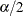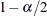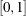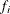The GLMSELECT Procedure

MODELAVERAGE Statement

• MODELAVERAGE <options>;

The MODELAVERAGE statement requests that model selection be repeated on resampled subsets of the input data. An average model is produced by averaging the parameter estimates of the selected models that are obtained for each resampled subset of the input data.

Table 48.9 summarizes the options available in the MODELAVERAGE statement.

Table 48.9: MODELAVERAGE Statement Options

Option

Description

Specifies lower and upper quantiles of the sample parameter

Displays model selection details

Specifies the number of samples used for the refit averaging

Performs a second round of model averaging

Specifies how to generate the samples taken from the training data

Uses only a subset of the selected models in forming the average model

Controls the displayed tables

The following options are available:

ALPHA=controls which lower and upper quantiles of the sample parameter estimates are displayed. The ALPHA= option also controls which quantiles of the predicted values are added to the output data set when the LOWER= and UPPER= options are specified in the OUTPUT statement. The lower and upper quantiles used areand, respectively. The value specified must lie in the interval. The default value is ALPHA=0.5.

DETAILS

requests that model selection details be displayed for each sample of the data. The level of detail shown is controlled by the DETAILS= option in the MODEL statement.

NSAMPLES=n

specifies the number of samples to be used. The default value is NSAMPLES=100.

REFIT <(refit-options)>

requests that a second round of model averaging, referred to as the refit averaging, be performed. Usually, the initial round of model averaging produces a model that contains a large number of effects. You can use the refit option to obtain a more parsimonious model. For each data sample in the refit, a least squares model is fit with no effect selection. The effects that are used in the refit depend on the results of the initial round of model averaging. If you do not specify any refit-options, then effects that are selected in at least twenty percent of the samples in the initial round of model averaging are used in the refit model average. The following refit-options are available:

BEST=n

specifies that the n most frequently selected effects in the initial round of model averaging be used in the refit averaging.

MINPCT=percent

specifies that the effects that are selected at least the specified percentage of times in the initial round of model averaging be used in the refit averaging.

NSAMPLES=n

specifies the number of samples to be used for the refit averaging. The default value is the number of samples used in the initial round of model averaging.

SAMPLING=SRS | URS <(sampling-options)>

specifies how the samples of the usable observations in the training data are generated. SAMPLING=SRS specifies simple random sampling in which samples are generated by randomly drawing without replacement. SAMPLING=URS specifies unrestricted random sampling in which samples are generated by randomly drawing with replacement. Model averaging with samples drawn without replacement corresponds to the bootstrap methodology. The default is SAMPLING=URS. If you specify a frequency variable by using a FREQ statement, then the ith observation is sampledtimes, whereis the frequency of the ith observation.

You can specify one of the following sampling-options:

PERCENT=percent

specifies the percentage of the training data that is used in each sample. The default value is 75% for SAMPLING=SRS and 100% for SAMPLING=URS.

SIZE=n

specifies the sum of frequencies in each sample.

SUBSET(subset-options)

specifies that only a subset of the selected models be used in forming the average model and producing predicted values. The following subset-options are available:

BEST=n

specifies that only the best n models be used, where the model ranking criterion used is the frequency score. See the section Model Selection Frequencies and Frequency Scores for the definition of the frequency score. If multiple models with the same frequency score correspond to the nth best model, then all these tied models are used in forming the average model and producing predicted values.

MINMODELFREQ=freq

specifies that only models that are selected at least freq times be used in forming the average model and producing predicted values.

TABLES <(ONLY)> <=table-request <(options)>>
TABLES <(ONLY)> <= (table-request <(options)> <... table-request <(options)>>)>

controls the displayed output that is produced in the initial round of model averaging. By default, the following tables are produced:

EFFECTSELECTPCT

displays the percentage of times that effects appear in the selected models.

MODELSELECTFREQ

displays the frequency with which models are selected.

AVGPARMEST

displays the mean, standard deviation, and quantiles of the parameter estimates of the parameters that appear in the selected models.

When you specify only one table-request, you can omit the outer parentheses. Here are some examples:

tables=none
tables=(all parmest(minpct=10))
tables(only)=effectselectpct(order=model minpct=15)


The following table-request options are available:

ALL

requests that all model averaging output tables be produced. You can specify other options with ALL; for example, to request all tables and to require that effects are displayed in decreasing order of selection frequency in the EffectSelectPct table, specify TABLES=(ALL EFFECTSELECTPCT(ORDER=DESCENDING)).

EFFECTSELECTPCT <(effectSelectPct-options)>

specifies how the effects in the EffectSelectPct table are displayed. The following effectSelectPct-options are available:

ALL

specifies that effects that appear in the selected model for any sample be displayed.

MINPCT=percent

specifies that the effects displayed must appear in the selected model for at least the specified percentage of the samples. By default, this table includes effects that appear in at least twenty percent of the selected models. The MINPCT= option is ignored if you also specify the ALL option as a effectSelectPct-option.

ORDER=ASCENDING | DESCENDING | MODEL

specifies the order in which the effects are displayed. ORDER=MODEL specifies that effects be displayed in the order in which they appear in the MODEL statement. ORDER= ASCENDING | DESCENDING specifies that the effects be displayed in ascending or descending order of their selection frequency.

MODELSELECTFREQ <(modelSelectFreq-options)>

specifies how the models in the ModelSelectFreq table are displayed. The following modelSelectFreq-options are available:

ALL

specifies that all selected models be displayed in the ModelSelectFreq table.

BEST=n

specifies that only the best n models be displayed, where the model ranking criterion used is the frequency score. See the section Model Selection Frequencies and Frequency Scores for the definition of the frequency score. The default value is BEST=20. The BEST= option is ignored if you also specify the ALL option as a modelSelectFreq-option.

ONLY

suppresses the default output. If you specify the ONLY option within parentheses after the TABLES option, then only the tables specifically requested are produced.

PARMEST <(parmEst-options)>

specifies how the parameters in the AvgParmEst table are displayed. The following parmEst-options are available:

ALL

specifies that parameters that are nonzero in the selected model for any sample be displayed.

MINPCT=percent

specifies that the parameters displayed must have nonzero estimates in the selected model for at least the specified percentage of the samples. By default, this table includes parameters that appear in at least twenty percent of the selected models. The MINPCT= option is ignored if you also specify the ALL option as a parmEst option.

NONZEROPARMS

specifies that for each parameter, the sample that is used to compute the estimate mean, standard deviation, and quantiles consist of just the nonzero values of that parameter in the selected models. If you do not specify the NONZEROPARMS option, then parameters that do not appear in a selected model are assigned the value zero in that model and these zero values are retained when computing the estimate means, standard deviations, and quantiles.

ORDER=ASCENDING | DESCENDING | MODEL

specifies the order in which the effects are displayed. ORDER=MODEL specifies that effects are displayed in the order in which they appear in the MODEL statement. ORDER=ASCENDING | DESCENDING specifies that the effects are displayed in ascending or descending order of their selection frequency.